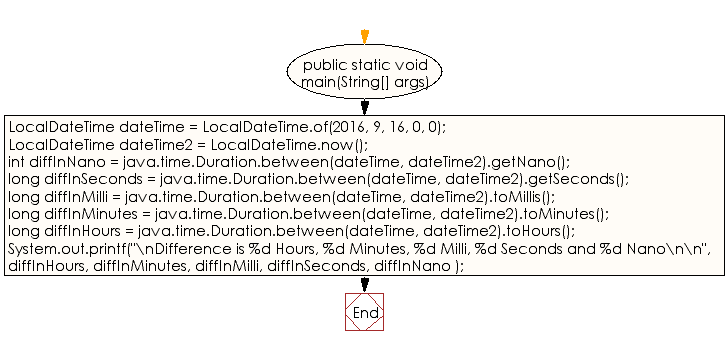﻿ Java exercises: Compute the difference between two dates (Hours, minutes, milli, seconds and nano) - w3resource# Java DateTime, Calendar Exercises: Compute the difference between two dates (Hours, minutes, milli, seconds and nano)

## Java DateTime, Calendar: Exercise-31 with Solution

Write a Java program to compute the difference between two dates (Hours, minutes, milli, seconds and nano).

Sample Solution:

Java Code:

``````import java.time.*;
import java.util.*;

public class Exercise31 {
public static void main(String[] args)
{
LocalDateTime dateTime = LocalDateTime.of(2016, 9, 16, 0, 0);
LocalDateTime dateTime2 = LocalDateTime.now();
int diffInNano = java.time.Duration.between(dateTime, dateTime2).getNano();
long diffInSeconds = java.time.Duration.between(dateTime, dateTime2).getSeconds();
long diffInMilli = java.time.Duration.between(dateTime, dateTime2).toMillis();
long diffInMinutes = java.time.Duration.between(dateTime, dateTime2).toMinutes();
long diffInHours = java.time.Duration.between(dateTime, dateTime2).toHours();
System.out.printf("\nDifference is %d Hours, %d Minutes, %d Milli, %d Seconds and %d Nano\n\n",
diffInHours, diffInMinutes, diffInMilli, diffInSeconds, diffInNano );

}
}
```
```

Sample Output:

```Difference is 6686 Hours, 401180 Minutes, 24070844780 Milli, 24070844 Seconds and 780000000 Nano
```

N.B.: The result may varry for your system date and time.

Flowchart:Java Code Editor:

Improve this sample solution and post your code through Disqus

What is the difficulty level of this exercise?

﻿

## Java: Tips of the Day

Directory Content:

Java allows you to get the names of all subdirectories and files in a folder as an array, which can then be sequentially viewed.

```import java.io.*;

public class ListContents {
public static void main(String[] args) {
File file = new File("//home//user//Documents/");
String[] files = file.list();

System.out.println("Listing contents of " + file.getPath());
for(int i=0 ; i < files.length ; i++)
{
System.out.println(files[i]);
}
}
}
```